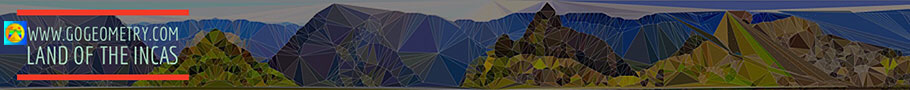# Geometría Plana: Puntos Colineales, Teoremas y ProblemasTeoremas y Problemas. Problema de Geometría 896 Triangulo, Ortocentro, Alturas, Puntos Medios, Diámetros, Circunferencias Secantes, Puntos Colineales. GeoGebra, Geometría Dinámica: Problema de Geometria 895 HTML5, Animación interactiva para iPad, Nexus y otras tabletas. Teorema de la Línea de Lemoine Puntos colineales. GeoGebra, Geometría Dinámica: Recta de Lemoine de un Triangulo . HTML5, Animación interactiva para iPad, Nexus y otras tabletas. Circulo de los Nueve Puntos: Spanish version. Recta de Euler, Puntos Colineales Interactive illustration. Pappus Theorem. Dynamic Geometry. Step-by-Step construction, Manipulation, and animation. Collinear Points. Interactive Gergonne Line and Nobbs Points. Dynamic Geometry. Step-by-Step construction, Manipulation, and animation. Collinear Points. Interactive Simson Line. Dynamic Geometry. Step-by-Step construction, Manipulation, and animation. Collinear Points. Sawayama -Thebault's theorem Collinear Points. Monge & d'Alembert Three Circles Theorem II with Dynamic Geometry. You can alter the geometric construction dynamically in order to test and prove (or disproved) conjectures and gain mathematical insight that is less readily available with static drawings by hand. Collinear Points. Monge & d'Alembert Three Circles Theorem I with Dynamic Geometry. You can alter the geometric construction dynamically in order to test and prove (or disproved) conjectures and gain mathematical insight that is less readily available with static drawings by hand. Collinear Points. Triangle with the bisectors of the exterior angles. Collinearity. Key concept: Menelaus Theorem. Collinear Points Complete Quadrilateral: Ortholine-Steiner Line. Step-by-Step construction, Manipulation, and animation Collinear Points. Triangle and Squares Theorem 1: with Interactive Geometry Software Step-by-Step construction, Manipulation, and animation. Collinear Points. Newton's Theorem, Newton-Gauss Line: Complete quadrilateral theorem. Using TracenPoche Dynamic Geometry Software, Online Step-by-Step construction, manipulation, and animation. Collinear Points. Schiffler Point: Four Euler Lines with interactive animation and manipulation. Collinear Points. Newton's Theorem: Newton's Line. Circumscribed quadrilateral, midpoints of diagonals, center of the circle inscribed. Collinear Points. Equilic Quadrilateral. Collinear Points. Geometry Problem 723. Squares, Circumscribed Circles, Collinear points. Geometry Problem 722. Squares, Circumscribed Circles, Collinear points. Geometry Problem 721. Triangle, Orthocenter, Circumcircle, Altitude, Collinear points. Geometry Problem 710. Parallelogram, Midpoint, Diagonal, Collinear Points, Metric Relations, Triangle, Similarity, Congruence. Geometry Problem 678. Triangle, Simson Line, Circumcircle, Tangent, Parallel, Perpendicular, Collinear Points. Geometry Problem 675. Intersecting Circles, Secant, 45 and 90 Degrees, Collinear Points. Geometry Problem 669. Triangle, Circumcircle, Incenter, Midpoint, Collinear points, Mind Map. Geometry Problem 659. Parallelogram, Parallel Lines, Collinear Points. Geometry Problem 632. Triangle, Interior and Exterior Angle Bisectors, Midpoints, Collinear Points. Geometry Problem 631. Triangle, Exterior Angle Bisectors, Collinear Points. Geometry Problem 613 Altitude of a Triangle, Perpendicular, Collinear Points. Geometry Problem 612 Altitude of a Triangle, Perpendicular, Congruence. Geometry Problem 611 Altitude of a Triangle, Perpendicular, Collinear Points, Parallel. Geometry Problem 609 Triangle, Perpendiculars, 90 Degrees, Collinearity. Geometry Problem 593. Triangle, Circumcircle, Collinear Points. Problem 570. Square, Center, Right Triangles, Congruence, Collinearity. Problem 567. Right triangle, Incircle, Excircle, Collinear tangency points. Problem 523. Tangent Circles, Diameter Perpendicular, Collinearity. Problem 486. Tangent circles, Chord, Arc, Midpoint, Collinearity, Congruence. Proposed Problem 456. Three tangent circles, Center, Chord, Secant, Collinear. Proposed Problem 393. Triangle, Orthocenter, Circumcircles, Congruence, Collinear. Proposed Problem 392. Triangle, Parallel lines, Concurrent lines. Proposed Problem 391. Triangle, Parallel lines, Collinear points. Proposed Problem 390. Triangle, Parallel lines, Collinear points. Proposed Problem 323. Triangle, Incenter, Circumcenter, Equal circles, Collinearity. Proposed Problem 321. Triangle, Incenter, Excircle, Midpoint of the Altitude, Collinearity. Proposed Problem 320. Triangle, Circumcircle, Incenter, Excenter, Collinear points. Proposed Problem 318. A Scalene triangle, Altitudes, Collinear points. Proposed Problem 319. Triangle, Altitudes, Perpendiculars, Collinear points. Proposed Problem 133. Triangle, Interior and Exterior Angle Bisectors, Collinear Points.

 Home | Search | Geometría | Líneas | Email | Publicar un comentario | by Antonio Gutierrez